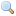# Module Int32

`module Int32: `sig` .. `end``
32-bit integers.

This module provides operations on the type `int32` of signed 32-bit integers. Unlike the built-in `int` type, the type `int32` is guaranteed to be exactly 32-bit wide on all platforms. All arithmetic operations over `int32` are taken modulo 232.

Performance notice: values of type `int32` occupy more memory space than values of type `int`, and arithmetic operations on `int32` are generally slower than those on `int`. Use `int32` only when the application requires exact 32-bit arithmetic.

`val zero : `int32``
The 32-bit integer 0.
`val one : `int32``
The 32-bit integer 1.
`val minus_one : `int32``
The 32-bit integer -1.
`val neg : `int32 -> int32``
Unary negation.
`val add : `int32 -> int32 -> int32``
`val sub : `int32 -> int32 -> int32``
Subtraction.
`val mul : `int32 -> int32 -> int32``
Multiplication.
`val div : `int32 -> int32 -> int32``
Integer division. Raise `Division_by_zero` if the second argument is zero. This division rounds the real quotient of its arguments towards zero, as specified for `Pervasives.(/)`.
`val rem : `int32 -> int32 -> int32``
Integer remainder. If `y` is not zero, the result of `Int32.rem x y` satisfies the following property: `x = Int32.add (Int32.mul (Int32.div x y) y) (Int32.rem x y)`. If `y = 0`, `Int32.rem x y` raises `Division_by_zero`.
`val succ : `int32 -> int32``
Successor. `Int32.succ x` is `Int32.add x Int32.one`.
`val pred : `int32 -> int32``
Predecessor. `Int32.pred x` is `Int32.sub x Int32.one`.
`val abs : `int32 -> int32``
Return the absolute value of its argument.
`val max_int : `int32``
The greatest representable 32-bit integer, 231 - 1.
`val min_int : `int32``
The smallest representable 32-bit integer, -231.
`val logand : `int32 -> int32 -> int32``
Bitwise logical and.
`val logor : `int32 -> int32 -> int32``
Bitwise logical or.
`val logxor : `int32 -> int32 -> int32``
Bitwise logical exclusive or.
`val lognot : `int32 -> int32``
Bitwise logical negation
`val shift_left : `int32 -> int -> int32``
`Int32.shift_left x y` shifts `x` to the left by `y` bits. The result is unspecified if `y < 0` or `y >= 32`.
`val shift_right : `int32 -> int -> int32``
`Int32.shift_right x y` shifts `x` to the right by `y` bits. This is an arithmetic shift: the sign bit of `x` is replicated and inserted in the vacated bits. The result is unspecified if `y < 0` or `y >= 32`.
`val shift_right_logical : `int32 -> int -> int32``
`Int32.shift_right_logical x y` shifts `x` to the right by `y` bits. This is a logical shift: zeroes are inserted in the vacated bits regardless of the sign of `x`. The result is unspecified if `y < 0` or `y >= 32`.
`val of_int : `int -> int32``
Convert the given integer (type `int`) to a 32-bit integer (type `int32`).
`val to_int : `int32 -> int``
Convert the given 32-bit integer (type `int32`) to an integer (type `int`). On 32-bit platforms, the 32-bit integer is taken modulo 231, i.e. the high-order bit is lost during the conversion. On 64-bit platforms, the conversion is exact.
`val of_float : `float -> int32``
Convert the given floating-point number to a 32-bit integer, discarding the fractional part (truncate towards 0). The result of the conversion is undefined if, after truncation, the number is outside the range [`Int32.min_int`, `Int32.max_int`].
`val to_float : `int32 -> float``
Convert the given 32-bit integer to a floating-point number.
`val of_string : `string -> int32``
Convert the given string to a 32-bit integer. The string is read in decimal (by default) or in hexadecimal, octal or binary if the string begins with `0x`, `0o` or `0b` respectively. Raise `Failure "int_of_string"` if the given string is not a valid representation of an integer, or if the integer represented exceeds the range of integers representable in type `int32`.
`val to_string : `int32 -> string``
Return the string representation of its argument, in signed decimal.
`val bits_of_float : `float -> int32``
Return the internal representation of the given float according to the IEEE 754 floating-point ``single format'' bit layout. Bit 31 of the result represents the sign of the float; bits 30 to 23 represent the (biased) exponent; bits 22 to 0 represent the mantissa.
`val float_of_bits : `int32 -> float``
Return the floating-point number whose internal representation, according to the IEEE 754 floating-point ``single format'' bit layout, is the given `int32`.
`type t = `int32` `
An alias for the type of 32-bit integers.
`val compare : `t -> t -> int``
The comparison function for 32-bit integers, with the same specification as `Pervasives.compare`. Along with the type `t`, this function `compare` allows the module `Int32` to be passed as argument to the functors `Set.Make` and `Map.Make`.